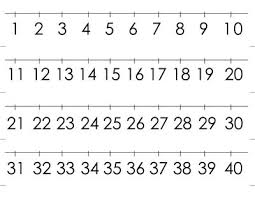# Interested 7090

We call a natural number N bombastic if it contains no zero in its notation and if no smaller natural number has the same product of digits as the number N. Charles first became interested in bombastic prime numbers and claimed that there were not many of them.
List all two-digit bombastic primes.

Then Charles chose one bombastic number and told us that it contained the digit three and that only one of his other digits was even. What even digit could it be?

b1 =  29
b2 =  37
b3 =  47
b4 =  59
b5 =  67
b6 =  79
b7 =  89
x1 =  8

### Step-by-step explanation:Did you find an error or inaccuracy? Feel free to write us. Thank you!